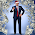## Monday, June 22, 2015

### Final Solution for Proton and Electron - Schrödinger's Wave Equation

See:
...for Complete Solution for Proton and Electron - Schrödinger's Wave Equation

Phase conjugate solutions to Schrödinger's Wave Equations are what is produced and the electron and proton are the opposite phases (180 degrees out of phase).  This is the standard method of solving these types of equations, and as in Quantum Field Theory (QFT) it is well known about the dagger notation of an operation to get a measurement, for example, a real result or a measurement is always a matrix product of two phase conjugate(dagger conjugate), spacetime (k-momentum space, time), thus any real manifestation will be the result of this type of product conjugate.

And of course complex conjugates are used in Maxwell's equations. I use complex conjugates all the time in my electrical engineering work.   Because Maxwell is always lurking in the background.  We will add more on our march for a complete theory and description.

These solutions will be just like Dan Winter's phase-conjugate solutions.

And The Electric Universe People are correct with their solutions as well... ...this is a unification theory, oneness.

Mark Eric Rohrbaugh and Lyz Starwalker
©2015 Mark Eric Rohrbaugh ©2015 Lyz Starwalker

1.The SIMULTANEOUS solution of the wave equation for both proton and electron.

2.since everyone speaks a different language, I must explain from what perspective to look at this. Note, there are MANY solutions that come from this. MANY. Thank you Lyz.

3.Anybody who deeply studies this stuff relies on Schrodinger's wave equation. These three ratios that Lyz and I solved for are the three that are needed for a complete solution. The other one is one.

4.I guess it's tough when one is given an answer and doesn't know what the question was. Hope this explanation helps in the understanding of its significance.
The question was, using Schrodinger Wave Equation, what is the solution to the proton mass and what is the solution for the electron mass, using whatever proven relationships available? and this is the solution

5.and of course complex conjugates are used in Maxwell's equations. I use complex conjugates all the time in my electrical engineering work.

6.Maxwell is ALWAYS lurking in the background, so you cannot forget the electric universe people!

7.orthogonally is how to extract more info from these equations. There's a lot more to come.

Simulations, correlations, and All.

Watch the water. 🦆